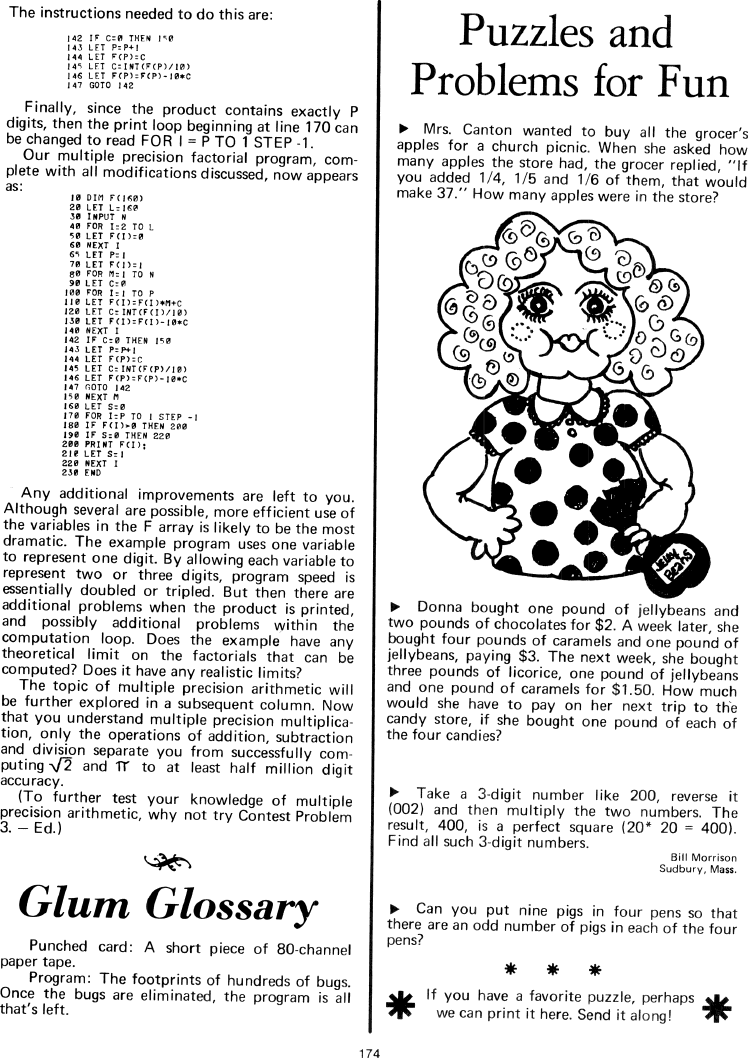The Best of Creative Computing Volume 1 (published 1976)

 `Page 174 << PREVIOUS >> NEXT Jump to page: Go to contents Go to thumbnails`

Puzzles and Problems for Fun (math puzzles)```The instructions needed to do this are:

|42 IF C=0 THEN l<0
|45 LET P=P l
|44 LET F(P)=C
|45 LET C=INT(F(P)/10)
|46 LET F(P)=F(P)-10*C
|47 GOTO |42

Finally, since the product contains exactly P digits, then the print loop
beginning at line 170 can be changed to read FOR I = P TO 1 STEP -1.

Our multiple precision factorial program, complete with all modifications
discussed, now appears as:

10 DIM F(160)
20 LET L=l60
30 INPUT N
40 FOR I=2 TO L
50 LET F(I)=0
60 NEXT I
65 LET P=1
70 LET F(l)=1
80 FOR M=1 TO N
90 LET C=0
100 FOR I=1 TO P
110 LET F(I)=F(I)*M C
120 LET C:INT(F(I)/l0)
l30 LET F(I)=F(I)-10*C
l40 NEXT I
142 IE C=0 THEN 150
143 LET P=P 1
144 LET F(P)=C
145 LET C:lNT(F(P)/l0)
146 LET F(P)=F(P)-10*C
147 GOTO 142
150 NEXT M
160 LET S=0
170 FOR I=P T0 1 STEP -1
180 IF F(I)>0 THEN 200
190 IF S=0 THEN 220
200 PRINT F(I):

210 LET S=1
220 NEXT I
230 END

Any additional improvements are left to you. Although several are possible, more
efficient use of the variables in the F array is likely to be the most dramatic.
The example program uses one variable
to represent one digit. By allowing each variable to represent two or three
digits, program speed is
essentially doubled or tripled. But then there are additional problems when the
product is printed,
and possibly additional problems within the computation loop. Does the example
have any theoretical limit on the factorials that can be computed? Does it have
any realistic limits?

The topic of multiple precision arithmetic will be further explored in a
subsequent column. Now that you understand multiple precision multiplication,
only the operations of addition, subtraction and division separate you from
successfully computing SQR(2) and (PI) to at least half million digit
accuracy.

(To further test your knowledge of multiple precision arithmetic, why not try
Contest Problem 3. - Ed.)

***

Glum Glossary

Punched card: A short piece of 80-channel paper tape.

Program: The footprints of hundreds of bugs. Once the bugs are eliminated, the
program is all that's left.

***

Puzzles and Problems for Fun

Mrs. Canton wanted to buy all the grocer's apples for a church picnic. When she
1/5 and 1/6 of them, that would make 37." How many apples were in the store?

[image]

Donna bought one pound of jellybeans and two pounds of chocolates for \$2. A week
later, she bought four pounds of caramels and one pound of jellybeans, paying
\$3. The next week, she bought three pounds of licorice, one pound of jellybeans
and one pound of caramels for \$1.50. How much would she have to pay on her next
trip to the candy store, if she bought one pound of each of the four candies?

Take a 3-digit number like 200, reverse it (002) and then multiply the two
numbers. The result, 400, is a perfect square (20*20 = 400). Find all such
3-digit numbers.

Bill Morrison
Sudbury, Mass.

Can you put nine pigs in four pens so that there are an odd number of pigs in
each of the four pens?

***
If you have a favorite puzzle, perhaps we can print it here. Send it along!

Page 174        << PREVIOUS        >> NEXT        Jump to page:                Go to contents        Go to thumbnails

<!--